Pattern Completion Quiz I, Non Verbal Reasoning

# Pattern Completion Quiz I, Non Verbal Reasoning - Mechanical Engineering

Test Description

## 25 Questions MCQ Test General Aptitude for GATE - Pattern Completion Quiz I, Non Verbal Reasoning

Pattern Completion Quiz I, Non Verbal Reasoning for Mechanical Engineering 2023 is part of General Aptitude for GATE preparation. The Pattern Completion Quiz I, Non Verbal Reasoning questions and answers have been prepared according to the Mechanical Engineering exam syllabus.The Pattern Completion Quiz I, Non Verbal Reasoning MCQs are made for Mechanical Engineering 2023 Exam. Find important definitions, questions, notes, meanings, examples, exercises, MCQs and online tests for Pattern Completion Quiz I, Non Verbal Reasoning below.
Solutions of Pattern Completion Quiz I, Non Verbal Reasoning questions in English are available as part of our General Aptitude for GATE for Mechanical Engineering & Pattern Completion Quiz I, Non Verbal Reasoning solutions in Hindi for General Aptitude for GATE course. Download more important topics, notes, lectures and mock test series for Mechanical Engineering Exam by signing up for free. Attempt Pattern Completion Quiz I, Non Verbal Reasoning | 25 questions in 50 minutes | Mock test for Mechanical Engineering preparation | Free important questions MCQ to study General Aptitude for GATE for Mechanical Engineering Exam | Download free PDF with solutions
 1 Crore+ students have signed up on EduRev. Have you?
Pattern Completion Quiz I, Non Verbal Reasoning - Question 1

### Directions to Solve In each of the following questions, select a figure from amongst the four alternatives, which when placed in the blank space of figure (X) would complete the pattern. Question - Identify the figure that completes the pattern.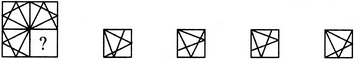(X)                 (1)             (2)              (3)             (4)

Detailed Solution for Pattern Completion Quiz I, Non Verbal Reasoning - Question 1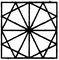Pattern Completion Quiz I, Non Verbal Reasoning - Question 2

### Directions to Solve In each of the following questions, select a figure from amongst the four alternatives, which when placed in the blank space of figure (X) would complete the pattern. Question - Identify the figure that completes the pattern.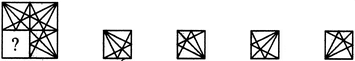(X)                 (1)             (2)              (3)             (4)

Detailed Solution for Pattern Completion Quiz I, Non Verbal Reasoning - Question 2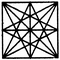Pattern Completion Quiz I, Non Verbal Reasoning - Question 3

### Directions to Solve In each of the following questions, select a figure from amongst the four alternatives, which when placed in the blank space of figure (X) would complete the pattern. Question - Identify the figure that completes the pattern.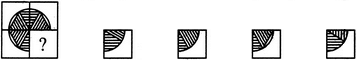(X)                 (1)             (2)              (3)             (4)

Detailed Solution for Pattern Completion Quiz I, Non Verbal Reasoning - Question 3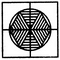Pattern Completion Quiz I, Non Verbal Reasoning - Question 4

In each of the following questions, select a figure from amongst the four alternatives, which when placed in the blank space of figure (X) would complete the pattern.

Question -

Identify the figure that completes the pattern.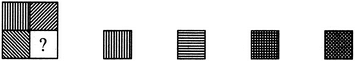(X)                 (1)             (2)              (3)             (4)
Detailed Solution for Pattern Completion Quiz I, Non Verbal Reasoning - Question 4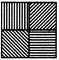Pattern Completion Quiz I, Non Verbal Reasoning - Question 5

In each of the following questions, select a figure from amongst the four alternatives, which when placed in the blank space of figure (X) would complete the pattern.

Question -

Identify the figure that completes the pattern.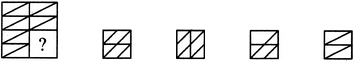(X)                 (1)             (2)              (3)             (4)
Detailed Solution for Pattern Completion Quiz I, Non Verbal Reasoning - Question 5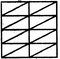Pattern Completion Quiz I, Non Verbal Reasoning - Question 6

In each of the following questions, select a figure from amongst the four alternatives, which when placed in the blank space of figure (X) would complete the pattern.

Question -

Identify the figure that completes the pattern.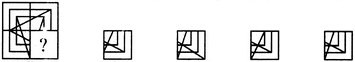(X)                 (1)             (2)              (3)             (4)
Detailed Solution for Pattern Completion Quiz I, Non Verbal Reasoning - Question 6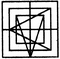Pattern Completion Quiz I, Non Verbal Reasoning - Question 7

In each of the following questions, select a figure from amongst the four alternatives, which when placed in the blank space of figure (X) would complete the pattern.

Question -

Identify the figure that completes the pattern.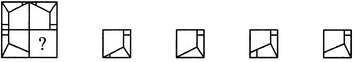(X)                 (1)             (2)              (3)             (4)
Detailed Solution for Pattern Completion Quiz I, Non Verbal Reasoning - Question 7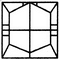Pattern Completion Quiz I, Non Verbal Reasoning - Question 8

In each of the following questions, select a figure from amongst the four alternatives, which when placed in the blank space of figure (X) would complete the pattern.

Question -

Identify the figure that completes the pattern.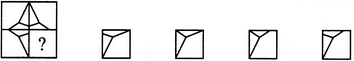(X)                 (1)             (2)              (3)             (4)
Detailed Solution for Pattern Completion Quiz I, Non Verbal Reasoning - Question 8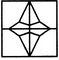Pattern Completion Quiz I, Non Verbal Reasoning - Question 9

In each of the following questions, select a figure from amongst the four alternatives, which when placed in the blank space of figure (X) would complete the pattern.

Question -

Identify the figure that completes the pattern.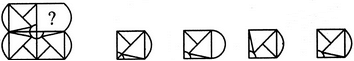(X)                 (1)             (2)              (3)             (4)
Detailed Solution for Pattern Completion Quiz I, Non Verbal Reasoning - Question 9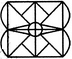Pattern Completion Quiz I, Non Verbal Reasoning - Question 10

In each of the following questions, select a figure from amongst the four alternatives, which when placed in the blank space of figure (X) would complete the pattern.

Question -

Identify the figure that completes the pattern.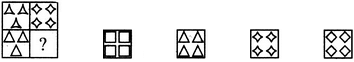(X)                 (1)             (2)              (3)             (4)
Detailed Solution for Pattern Completion Quiz I, Non Verbal Reasoning - Question 10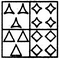Pattern Completion Quiz I, Non Verbal Reasoning - Question 11

In each of the following questions, select a figure from amongst the four alternatives, which when placed in the blank space of figure (X) would complete the pattern.

Question -

Identify the figure that completes the pattern.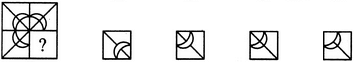(X)                 (1)             (2)              (3)             (4)
Detailed Solution for Pattern Completion Quiz I, Non Verbal Reasoning - Question 11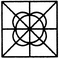Pattern Completion Quiz I, Non Verbal Reasoning - Question 12

In each of the following questions, select a figure from amongst the four alternatives, which when placed in the blank space of figure (X) would complete the pattern.

Question -

Identify the figure that completes the pattern.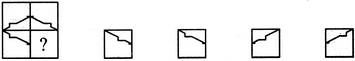(X)                 (1)             (2)              (3)             (4)
Detailed Solution for Pattern Completion Quiz I, Non Verbal Reasoning - Question 12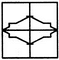Pattern Completion Quiz I, Non Verbal Reasoning - Question 13

In each of the following questions, select a figure from amongst the four alternatives, which when placed in the blank space of figure (X) would complete the pattern.

Question -

Identify the figure that completes the pattern.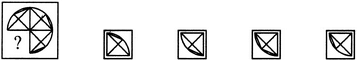(X)                 (1)             (2)              (3)             (4)
Detailed Solution for Pattern Completion Quiz I, Non Verbal Reasoning - Question 13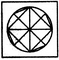Pattern Completion Quiz I, Non Verbal Reasoning - Question 14

Directions to Solve

In each of the following questions, select a figure from amongst the four alternatives, which when placed in the blank space of figure (X) would complete the pattern.

Question -

Identify the figure that completes the pattern.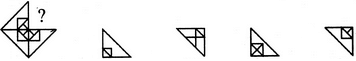(X)                 (1)             (2)              (3)             (4)

Detailed Solution for Pattern Completion Quiz I, Non Verbal Reasoning - Question 14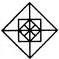Pattern Completion Quiz I, Non Verbal Reasoning - Question 15

In each of the following questions, select a figure from amongst the four alternatives, which when placed in the blank space of figure (X) would complete the pattern.

Question -

Identify the figure that completes the pattern.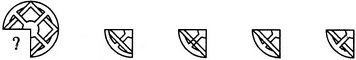(X)                 (1)             (2)              (3)             (4)
Detailed Solution for Pattern Completion Quiz I, Non Verbal Reasoning - Question 15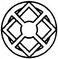Pattern Completion Quiz I, Non Verbal Reasoning - Question 16

In each of the following questions, select a figure from amongst the four alternatives, which when placed in the blank space of figure (X) would complete the pattern.

Question -

Identify the figure that completes the pattern.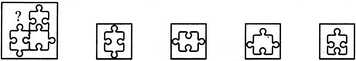(X)                 (1)             (2)              (3)             (4)
Detailed Solution for Pattern Completion Quiz I, Non Verbal Reasoning - Question 16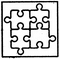Pattern Completion Quiz I, Non Verbal Reasoning - Question 17

In each of the following questions, select a figure from amongst the four alternatives, which when placed in the blank space of figure (X) would complete the pattern.

Question -

Identify the figure that completes the pattern.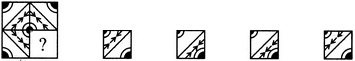(X)                 (1)             (2)              (3)             (4)
Detailed Solution for Pattern Completion Quiz I, Non Verbal Reasoning - Question 17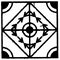Pattern Completion Quiz I, Non Verbal Reasoning - Question 18

In each of the following questions, select a figure from amongst the four alternatives, which when placed in the blank space of figure (X) would complete the pattern.

Question -

Identify the figure that completes the pattern.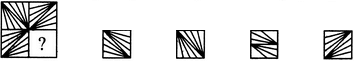(X)                 (1)             (2)              (3)             (4)
Detailed Solution for Pattern Completion Quiz I, Non Verbal Reasoning - Question 18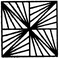Pattern Completion Quiz I, Non Verbal Reasoning - Question 19

In each of the following questions, select a figure from amongst the four alternatives, which when placed in the blank space of figure (X) would complete the pattern.

Question -

Identify the figure that completes the pattern.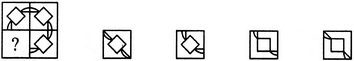(X)                 (1)             (2)              (3)             (4)
Detailed Solution for Pattern Completion Quiz I, Non Verbal Reasoning - Question 19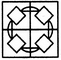Pattern Completion Quiz I, Non Verbal Reasoning - Question 20

In the following question, select a figure from amongst the four alternatives, which when placed in the blank space of fig. (X) would completer the pattern.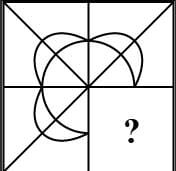Detailed Solution for Pattern Completion Quiz I, Non Verbal Reasoning - Question 20

Here, diagonals of  square.Hence there will be a straight line continuation from the line to second quadrant. Here, we have crescent shaped thing which is being rotated by an angle of 90 degree in clockwise direction. Hence, final figure will be combination of this shape and straight line making option C as correct answer.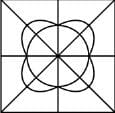Pattern Completion Quiz I, Non Verbal Reasoning - Question 21

In each of the following questions, select a figure from amongst the four alternatives, which when placed in the blank space of figure (X) would complete the pattern.

Question -

Identify the figure that completes the pattern.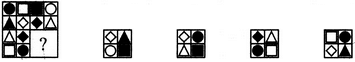(X)                 (1)             (2)              (3)             (4)
Detailed Solution for Pattern Completion Quiz I, Non Verbal Reasoning - Question 21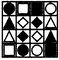Pattern Completion Quiz I, Non Verbal Reasoning - Question 22

In each of the following questions, select a figure from amongst the four alternatives, which when placed in the blank space of figure (X) would complete the pattern.

Question -

Identify the figure that completes the pattern.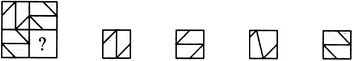(X)                 (1)             (2)              (3)             (4)
Detailed Solution for Pattern Completion Quiz I, Non Verbal Reasoning - Question 22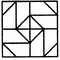Pattern Completion Quiz I, Non Verbal Reasoning - Question 23

In each of the following questions, select a figure from amongst the four alternatives, which when placed in the blank space of figure (X) would complete the pattern.

Question -

Identify the figure that completes the pattern.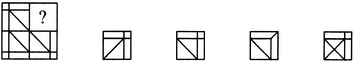(X)                 (1)             (2)              (3)             (4)
Detailed Solution for Pattern Completion Quiz I, Non Verbal Reasoning - Question 23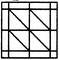Pattern Completion Quiz I, Non Verbal Reasoning - Question 24

In each of the following questions, select a figure from amongst the four alternatives, which when placed in the blank space of figure (X) would complete the pattern.

Question -

Identify the figure that completes the pattern.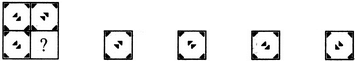(X)                 (1)             (2)              (3)             (4)
Detailed Solution for Pattern Completion Quiz I, Non Verbal Reasoning - Question 24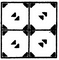Pattern Completion Quiz I, Non Verbal Reasoning - Question 25

In each of the following questions, select a figure from amongst the four alternatives, which when placed in the blank space of figure (X) would complete the pattern.

Question -

Identify the figure that completes the pattern.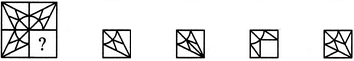(X)                 (1)             (2)              (3)             (4)
Detailed Solution for Pattern Completion Quiz I, Non Verbal Reasoning - Question 25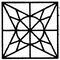## General Aptitude for GATE

84 videos|84 docs|108 tests
Information about Pattern Completion Quiz I, Non Verbal Reasoning Page
In this test you can find the Exam questions for Pattern Completion Quiz I, Non Verbal Reasoning solved & explained in the simplest way possible. Besides giving Questions and answers for Pattern Completion Quiz I, Non Verbal Reasoning, EduRev gives you an ample number of Online tests for practice

## General Aptitude for GATE

84 videos|84 docs|108 tests